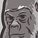Announcements
Applications are open for the 2024 Qlik Luminary Program. Apply by December 15 here.
cancel
Showing results for
Did you mean:Contributor III

## Reference Line - Average

Hello,

I have a bar chart and each bar is a customer and the height of the bar is a custom measure that calculates the % of sales (user selects a product group and can see what % of each customers sales are made up of that product group). The calculation limits the results to customers with at least \$5k in sales.

I am trying to create a reference line that shows the average across all of the customers. Not the total average (total product group sales / total sales) but the average of the % of sales across the customers.

My custom measure is:

if(sum({1<Year={"\$(=max(Year))"}>}
Sales)>5000,

sum({\$<Year={"\$(=max(Year))"}>}
Sales)
/
sum({<Year={"\$(=max(Year))"}, ProductGroup=>}
Sales)
,0)

I have tried wrapping the whole calculation in avg(aggr( but can't seem to make that work. Any ideas on how I can get the reference line calculation that I'm looking for?

Thank youMVP

Not sure, but may be you need this

```Avg(TOTAL Aggr(
If(Sum({1<Year = {"\$(=Max(Year))"}>} Sales) > 5000,
Sum({\$<Year = {"\$(=Max(Year))"}>} Sales)
/
Sum({<Year = {"\$(=Max(Year))"}, ProductGroup>} Sales)
, 0)
, Customer))```Tags
Community Browser